# Rasterizing Chromatograms

Version 1: Wednesday 13 June 2018This report describes an application of the 'rasterize' package for R. We rasterize (just) the line segments of two chromatograms in order to render one semi-transparently over the other. This also demonstrates, more generally, the value of having access to advanced graphical techniques within statistical graphics software.

## 1. The problem

A question on R-help (Ed Siefker, "How to alpha entire plot?", 2018-05-31) described a situation where two chromatograms, each consisting of lots of vertical lines, are to be overlaid. Because there is a lot of overlap, one chromatogram obscures the other. The following code and plot shows a representation of the problem; these are not chromatograms, but they share the essential graphical characteristics, i.e., lots of vertical line segments.

```EU <- data.frame(EuStockMarkets)
with(EU, plot(DAX, CAC, col="blue", type="h", ylim=c(0,6000)))
par(new=TRUE)
with(EU, plot(DAX, FTSE, col="red", type="h", ylim=c(0,6000)))
```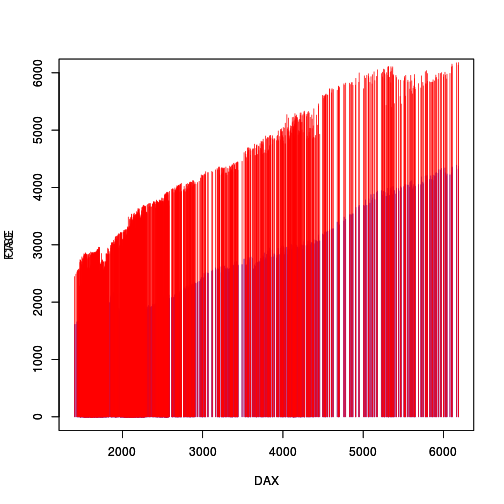A simple solution to this sort of overlap problem is to use semi-transparent colours to draw the vertical lines. The problem in this case is that the lines within a single chromatogram overlap with each other, so making each line semi-transparent still creates an opaque region if there are several lines from the same chromatogram overlapping each other. The following code and plot demonstrates this problem.

```col1 <- adjustcolor("blue", alpha=.3)
with(EU, plot(DAX, CAC, col=col1, type="h", ylim=c(0,6000)))
par(new=TRUE)
with(EU, plot(DAX, FTSE, col=col2, type="h", ylim=c(0,6000)))
```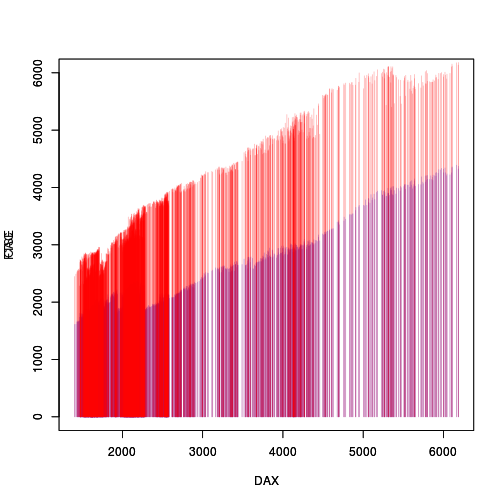The R-help question asked whether it is possible to draw the chromatogram lines with opaque colours and then make the entire set of lines semi-transparent (and then overlay the result). This report looks at implementing that solution using the 'rasterize' package (Murrell, 2018b, Murrell, 2018a) and the 'gridGraphics' package (Murrell and Wen, 2018) in R (R Core Team, 2018).

In brief, we will convert the plot to a 'grid' plot with 'gridGraphics' (Preparation: converting to 'grid'), rasterize the line segments with 'rasterize' (Rasterizing the chromatographs), and then adjust the semi-transparency of the rasterized line segments (Adding semi-transparency).

## 2. Preparation: converting to 'grid'

We start with the original plot, with its opaque colours so that the red line segments almost completely obscure the blue line segments.

```EU <- data.frame(EuStockMarkets)
with(EU, plot(DAX, CAC, col="blue", type="h", ylim=c(0,6000)))
par(new=TRUE)
with(EU, plot(DAX, FTSE, col="red", type="h", ylim=c(0,6000)))
```The 'rasterize' package works with 'grid' graphics output, so the first step is to convert the base graphics plot to a 'grid' one. This is achieved using `grid.echo` from the 'gridGraphics' package, as shown below (the resulting plot is visually identical to the original).

```library(gridGraphics)
grid.echo()
```## 3. Rasterizing the chromatographs

The next step is to rasterize the two sets of line segments. We can do this with the `grid.rasterize` function from the 'rasterize' package.

The first argument to this function is the name of a 'grid' grob that we want to rasterize, so we need to find out the names of the 'grid' grobs that represent the line segments. We also need to know the viewports that the line segments were drawn within because the rasterization has to occur within that viewport. The following code uses that `grid.ls` function to get us the information we need.

```grid.ls(viewports=TRUE, print=grobPathListing)
```
```  ROOT::graphics-root::graphics-inner::graphics-figure-1::graphics-plot-1-clip::graphics-window-1-1 | graphics-plot-1-spike-1
ROOT::graphics-root::graphics-inner::graphics-figure-1::graphics-plot-1::graphics-window-1-1      | graphics-plot-1-bottom-axis-line-1
ROOT::graphics-root::graphics-inner::graphics-figure-1::graphics-plot-1::graphics-window-1-1      | graphics-plot-1-bottom-axis-ticks-1
ROOT::graphics-root::graphics-inner::graphics-figure-1::graphics-plot-1::graphics-window-1-1      | graphics-plot-1-bottom-axis-labels-1
ROOT::graphics-root::graphics-inner::graphics-figure-1::graphics-plot-1::graphics-window-1-1      | graphics-plot-1-left-axis-line-1
ROOT::graphics-root::graphics-inner::graphics-figure-1::graphics-plot-1::graphics-window-1-1      | graphics-plot-1-left-axis-ticks-1
ROOT::graphics-root::graphics-inner::graphics-figure-1::graphics-plot-1::graphics-window-1-1      | graphics-plot-1-left-axis-labels-1
ROOT::graphics-root::graphics-inner::graphics-figure-1::graphics-plot-1                           | graphics-plot-1-box-1
ROOT::graphics-root::graphics-inner::graphics-figure-1-clip::graphics-plot-1                      | graphics-plot-1-xlab-1
ROOT::graphics-root::graphics-inner::graphics-figure-1-clip::graphics-plot-1                      | graphics-plot-1-ylab-1
ROOT::graphics-root::graphics-inner::graphics-figure-2::graphics-plot-2-clip::graphics-window-2-1 | graphics-plot-2-spike-1
ROOT::graphics-root::graphics-inner::graphics-figure-2::graphics-plot-2::graphics-window-2-1      | graphics-plot-2-bottom-axis-line-1
ROOT::graphics-root::graphics-inner::graphics-figure-2::graphics-plot-2::graphics-window-2-1      | graphics-plot-2-bottom-axis-ticks-1
ROOT::graphics-root::graphics-inner::graphics-figure-2::graphics-plot-2::graphics-window-2-1      | graphics-plot-2-bottom-axis-labels-1
ROOT::graphics-root::graphics-inner::graphics-figure-2::graphics-plot-2::graphics-window-2-1      | graphics-plot-2-left-axis-line-1
ROOT::graphics-root::graphics-inner::graphics-figure-2::graphics-plot-2::graphics-window-2-1      | graphics-plot-2-left-axis-ticks-1
ROOT::graphics-root::graphics-inner::graphics-figure-2::graphics-plot-2::graphics-window-2-1      | graphics-plot-2-left-axis-labels-1
ROOT::graphics-root::graphics-inner::graphics-figure-2::graphics-plot-2                           | graphics-plot-2-box-1
ROOT::graphics-root::graphics-inner::graphics-figure-2-clip::graphics-plot-2                      | graphics-plot-2-xlab-1
ROOT::graphics-root::graphics-inner::graphics-figure-2-clip::graphics-plot-2                      | graphics-plot-2-ylab-1
```

The line segments are represented by grobs called `graphics-plot-1-spike-1` and `graphics-plot-2-spike-1`, which are drawn within viewports called `graphics-window-1-1` and `graphics-window-2-1`, respectively.

```grid.get("graphics-plot-1-spike-1")
```
```  segments[graphics-plot-1-spike-1]
```

The following code navigates to the relevant viewports and rasterizes the two sets of line segments. Again, the resulting plot is very similar to the original plot, though the line segments may look a little fuzzy depending on the resolution we use for rasterization (and the size of the plot when viewed). We can adjust the rasterization resolution with the `res` argument to `grid.rasterize`.

```library(rasterize)
downViewport("graphics-window-1-1")
grid.rasterize("graphics-plot-1-spike-1")
upViewport(0)
downViewport("graphics-window-2-1")
grid.rasterize("graphics-plot-2-spike-1")
upViewport(0)
```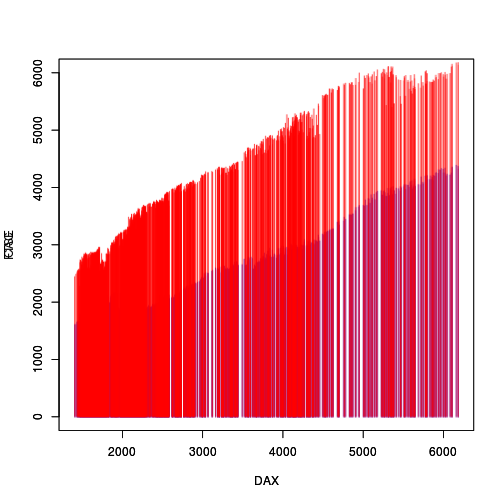The "spike" grobs are now raster grobs rather than line segment grobs.

```grid.get("graphics-plot-1-spike-1")
```
```  rastergrob[graphics-plot-1-spike-1]
```

The advantage of rasterizing the line segments is that we now have a collection of (non-overlapping) pixels, rather than lots of overlapping line segments. This means that we can modify the semi-transparency of the pixels and get a consistent semi-transparency across the entire chromatogram.

The following code extracts the matrix of pixels for each raster grob, adjusts the semi-transparency ("alpha channel") of each set of pixels, and edits each raster grob to replace the opaque pixels with the semi-transparent pixels.

```adjustAlpha <- function(grob) {
raster <- as.matrix(grid.get(grob)\$raster)
dim(newRaster) <- dim(raster)
grid.edit(grob, raster=as.raster(newRaster))
}
```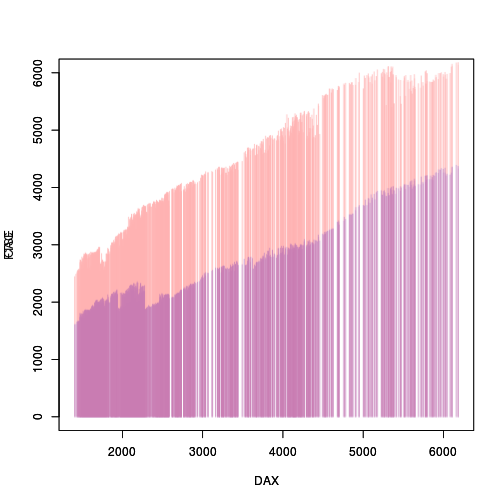As a final tweak, we can remove all of the decorations (axes and labels) from the second plot and modify the y-axis label.

```grid.remove(".+-plot-2-[^s].+", grep=TRUE, global=TRUE)
grid.edit("graphics-plot-1-ylab-1", label="CAC / FTSE")
```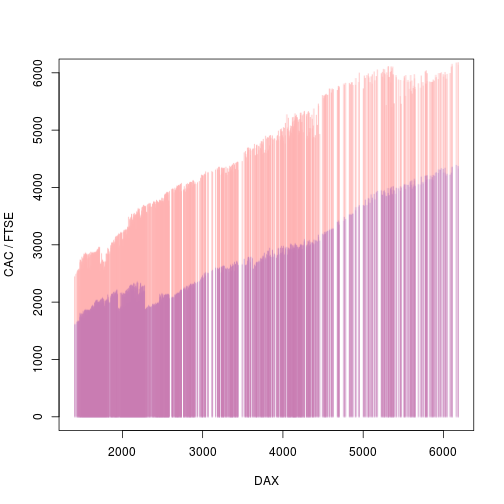## 5. Alternative solutions

The key idea in the solution outlined above is that we convert just some pieces of an image into raster components and then apply a modification just to those components. In effect, we draw the sets of line segments as separate images, but ones that are nicely aligned with the overall plot, and apply a modification to the separate images.

Another way that we could achieve the same result is to make use of filters from the 'gridSVG' package (Murrell and Potter, 2017). Again, the main idea is that we take a specific component of the plot and apply a modification just to that component.

The following code defines a "component transfer" filter with a transfer function that multiplies the alpha channel of its target by 0.3 (to make it semi-transparent).

```library(gridSVG)
reduceAlpha <- transferFunction("linear", slope=0.3)
filter <- feComponentTransfer(transfers=list(A=reduceAlpha))
```

The next code draws the initial chromatograms and converts to 'grid' as before.

```EU <- data.frame(EuStockMarkets)
with(EU, plot(DAX, CAC, col="blue", type="h", ylim=c(0,6000)))
par(new=TRUE)
with(EU, plot(DAX, FTSE, col="red", type="h", ylim=c(0,6000)))
library(gridGraphics)
grid.echo()
```

Now we apply the 'gridSVG' filters that we defined above to each of the sets of line segments (instead of rasterizing the line segments), then we export the result as an SVG file (which is shown below the code).

```grid.filter("graphics-plot-1-spike-1", filterEffect(filter))
grid.filter("graphics-plot-2-spike-1", filterEffect(filter))
grid.export("chromatograms.svg")
```One disadvantage of this approach is that it is quite slow to generate the file and the result is a much heftier file: about 1MB compared to approximately 20KB for all of the other PNG images in this report. This is because the image contains several thousand line segments (with a filter applied to them) rather than two raster images.

Another variation is to use the 'magick' package (Ooms, 2018) to perform the modifications of the raster grobs once we have rasterized the chromatograms. The advantage of this is that 'magick' offers many different raster manipulations.

For example, if we start the initial set up as before and rasterize the sets of line segments ...

```EU <- data.frame(EuStockMarkets)
with(EU, plot(DAX, CAC, col="blue", type="h", ylim=c(0,6000)))
par(new=TRUE)
with(EU, plot(DAX, FTSE, col="red", type="h", ylim=c(0,6000)))
library(gridGraphics)
grid.echo()
library(rasterize)
downViewport("graphics-window-1-1")
grid.rasterize("graphics-plot-1-spike-1")
upViewport(0)
downViewport("graphics-window-2-1")
grid.rasterize("graphics-plot-2-spike-1")
upViewport(0)
```

... the following code extracts the pixels from each raster grob and converts to 'magick' image objects.

```library(magick)
raster1 <- grid.get("graphics-plot-1-spike-1")\$raster
raster2 <- grid.get("graphics-plot-2-spike-1")\$raster
```

We can now apply all sorts of manipulations to those 'magick' images. The following code composites the two images with a "blend" operator so that the result is a 50/50 mixture of the two images.

```image3 <- image_composite(image1, image2, operator="blend", compose_args="50")
```

As a final step, we can remove one of the original raster grobs and replace the pixels in the other raster grob with the composited 'magick' image.

```grid.remove("graphics-plot-2-spike-1")
grid.edit("graphics-plot-1-spike-1", raster=as.raster(image3))
```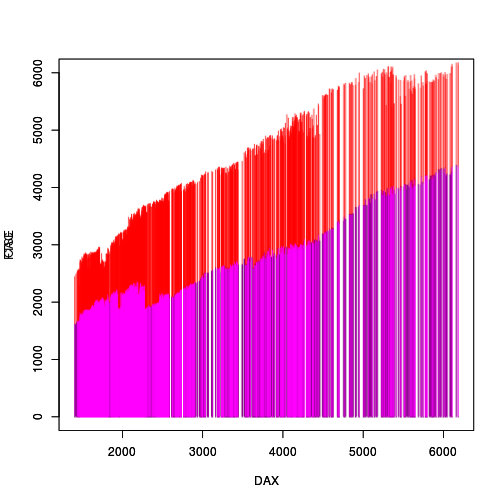Although it does not answer the original question directly, Boris Stiepe proposed a completely different solution based on discretizing the x-values of the chromatograms and then interleaving them. This solution has the nice property that the density of the chromatograms is retained.

## 6. Discussion

The 'rasterize' package was originally created to solve the problem of large file sizes (and slow rendering) when a vector format plot contains a large number of plotting symbols (for example). This report demonstrates that the ability to generate rasterized versions of plot components is also useful for performing raster operations on just specific components of a plot.

More generally, the example in this report provides a justification for providing access to more sophisticated graphical operations within statistical graphics software, through packages like 'rasterize', 'magick', and 'gridSVG'. Having access to more advanced graphical tools means that we can start thinking of doing different things and solving different problems.

## 7. Summary

Rasterizing specific components of a plot with the 'rasterize' package can be useful for applying raster transformations to the rasterized components. In this report, we used this approach to rasterize two sets of line segments and then modified the transparency of the resulting raster images so that they could be overlaid. The 'gridSVG' package and the 'magick' package can be used instead of or in combination with 'rasterize' to broaden the range of transformations that are possible.

## 8. Technical requirements

The examples and discussion in this document relate to version 0.1 of the 'rasterize' package.

This report was generated within a Docker container (see Resources section below).

## 9. Resources

• The raw source file for this report, a valid XML transformation of the source file, a 'knitr' document generated from the XML file, two R files and the bibtex file that are used to generate the table of contents and reference sections, two XSL files and an R file that are used to transform the XML to the 'knitr' document, and a Makefile that contains code for the other transformations and coordinates everything. These materials are also available on github.
• This report was generated within a Docker container. The Docker command to build the report is included in the Makefile above. The Docker image for the container is available from Docker Hub; alternatively, the image can be rebuilt from its Dockerfile.

## How to cite this document

Murrell, P. (2018). "Rasterizing Chromatograms" Technical Report 2018-06, Department of Statistics, The University of Auckland. [ bib ]

## 10. References

[Murrell, 2018a]
Murrell, P. (2018a). rasterize: Rasterize Graphical Output. R package version 0.1. [ bib ]
[Murrell, 2018b]
Murrell, P. (2018b). Selective raster graphics. Technical Report 2018-05, Department of Statistics, The University of Auckland. [ bib ]
[Murrell and Potter, 2017]
Murrell, P. and Potter, S. (2017). gridSVG: Export 'grid' Graphics as SVG. R package version 1.6-0. [ bib ]
[Murrell and Wen, 2018]
Murrell, P. and Wen, Z. (2018). gridGraphics: Redraw Base Graphics Using 'grid' Graphics. R package version 0.3-1. [ bib | http ]
[Ooms, 2018]
Ooms, J. (2018). magick: Advanced Graphics and Image-Processing in R. R package version 1.7. [ bib | http ]
[R Core Team, 2018]
R Core Team (2018). R: A Language and Environment for Statistical Computing. R Foundation for Statistical Computing, Vienna, Austria. [ bib | http ]